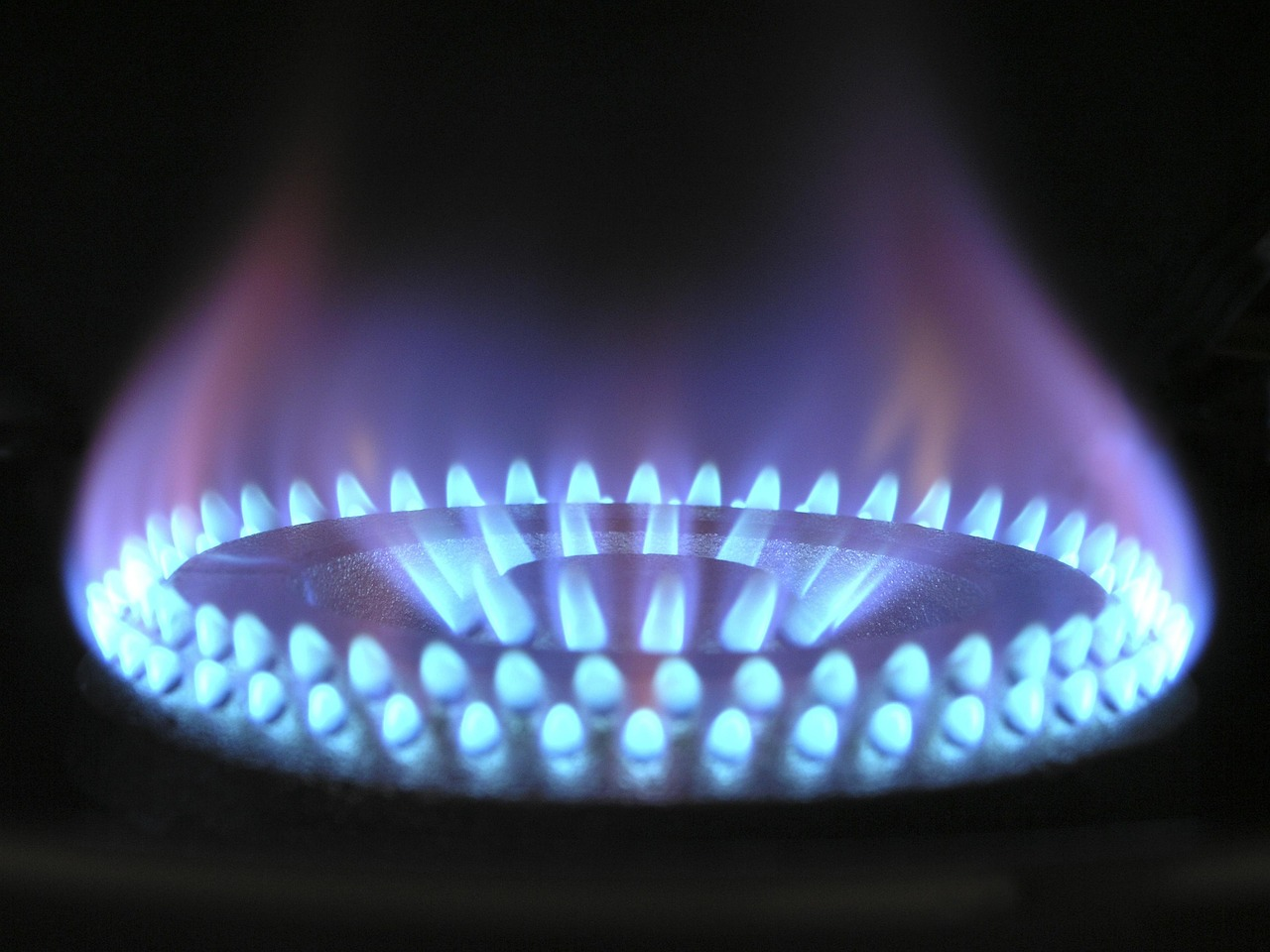📚

All Subjects

>

🧪

AP Chem

>

🔥

Unit 6

6.10 Multiple Choice Questions

AP Chemistry🧪

Bookmarked 6.5k • 251 resources
See Units

Multiple Choice Practice for Thermochemistry and Reaction Thermodynamics

Welcome to Unit 6 AP Chemistry Multiple Choice Questions! Grab some paper and a pencil 📄 to record your answers as you go. You can see how you did on the Unit 6 Practice Questions Answers and Review sheet once you're done. Don't worry, we have tons of resources available if you get stumped 😕 on a question. And if solo study is not your thing, join a group in Hours!
Not ready to take a quiz yet? Take a look at the Intro to Unit 6Resources:

Image courtesy of Pixabay

Facts about the test: The AP Chemistry exam has 60 multiple choice questions and you will be given 1 hour 30 minutes to complete the section. That means it should take you around 15 minutes to complete 10 questions. *The following questions were not written by College Board and, although they cover information outlined in the AP Chemistry Course and Exam Description, the formatting on the exam may be different.

1. Which of the following is defined by the measure of the average kinetic energy?
A. Potential Energy
B. Velocity
C. Heat
D. Temperature

2. What is the amount of energy is required to raise the temperature of 43 g of liquid water from 25°C to 55°C? Water's specific heat is 4.18 J/g°C.
A. 5.39 kJ
B. 5390 kJ
C. 4.49 kJ
D. 9.88 kJ

3. What is the amount of energy required to melt 7.2 g of water at 0°C? Water's enthalpy of fusion is 6.01 kJ/mol and water's enthalpy of vaporization is 40.7 kJ/mol.
A. 16.3 kJ
B. 43.3 kJ
C. 293 kJ
D. 2.40 kJ

4. When this reaction occurs, 75.0 kJ of energy is released. What mass of copper is produced?A. 0.588 g
B. 36.7 g
C. 110 g
D. 1.73 g

5. Which of the following statements is true regarding the following reaction?A. The reaction will cause the surrounding's temperature to increase because the reaction is exothermic.
B. The reaction will cause the surrounding's temperature to decrease because the reaction is exothermic.
C. The reaction will cause the surrounding's temperature to increase because the reaction is endothermic.
D. The reaction will cause the surrounding's temperature to decrease because the reaction is endothermic.

6. Determine the enthalpy of the reaction given the following data.A. 352.6 kJ/mol
B. -352.6 kJ/mol
C. 176.3 kJ/mol
D. -176.3 kJ/mol

7. Which of the following is an endothermic process?
A. Combusting methane
B. Freezing benzene
C. Condensing water
D. Melting wax

8. What is the enthalpy of reaction depicted in the reaction below?A. -299.4 kJ/mol
B. 149.7 kJ/mol
C. -149.7 kJ/mol
D. 299.4 kJ/mol

9. Which of the following process is exothermic?
A. CH4 (g) + 2O2(g) -> 2H2O(g) + CO2 (g)
B. H2O (l) -> H2O (g)
C. CO2 (s) -> CO2 (g)
D. CaCO3 (s) -> CaO (s) + CO2(g)

10. When hot piece of metal is placed into cold water, which of the following statements is true?
A. The final temperature for the metal will be hotter than the initial temperature of the metal at thermal equilibrium.
B. The final temperature of the metal will be cooler than the initial temperature of the water at thermal equilibrium.
C. The average kinetic energy of the water will increase while the average kinetic energy for the metal will decrease.
D. The average kinetic energy of the metal will increase while the average kinetic energy for the water will decrease.

11. A 25 g piece of metal required 227.5 J of energy to raise it by 10°C. What is the identity of the metal?
A. Iron (C=0.44 J/g°C)
B. Aluminum (C=0.91 J/g°C)
C. Copper (C=0.39 J/g°C)

12. How much heat is released when 5.00 g of propane (M=44.11g/mol) combusts? The heat of combustion for propane is -2220 kJ/mol.A. 11100 kJ
B. 252 kJ
C. 50.3 kJ
D. 84.0 kJ

13. Consider the following reaction. Which of the following statements is true?A. More energy is released when the bonds form than energy is required to break the bonds, because the reaction is exothermic.
B. More energy is released when the bonds form than energy is required to break the bonds, because the reaction is endothermic.
C. Less energy is released when the bonds form than energy is required to break the bonds, because the reaction is exothermic.
D. Less energy is released when the bonds form than energy is required to break the bonds, because the reaction is endothermic.

14. What is the final temperature of 15 g of nickel (C=0.44 J/g°C) that absorbs 240 J of energy at 15°C?
A. 36°C
B. -21°C
C. 22°C
D. 51°C

15. A student uses a calorimeter to determine the enthalpy of combustion of methane. Which of the following statements is true?
A. After the combustion the temperature of the calorimeter will decrease because combustion reactions are exothermic.
B. After the combustion the temperature of the calorimeter will increase because combustion reactions are exothermic.
C. After the combustion the temperature of the calorimeter will increase because combustion reactions are endothermic.
D. After the combustion the temperature of the calorimeter will decrease because combustion reactions are endothermic.

• 🤝Connect with other students studying AP Chem with Hours

Resources:Studying with Hours = the ultimate focus mode
Start a free study session
Browse Study Guides By Unit
📆Big Reviews: Finals & Exam Prep
✍️Free Response Questions
🧐Multiple Choice Questions
⚛️Unit 1: Atomic Structure and Properties
🤓Unit 2: Molecular and Ionic Compound Structures and Properties
🌀Unit 3: Intermolecular Forces and Properties
🧪Unit 4: Chemical Reactions
👟Unit 5: Kinetics
🔥Unit 6: Thermodynamics
⚖️Unit 7: Equilibrium
🍊Unit 8: Acids and Bases
🔋Unit 9: Applications of ThermodynamicsFREE AP chem Survival Pack + Cram Chart PDF